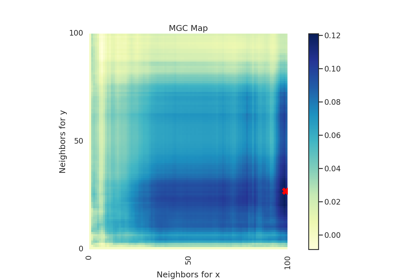# MaxMargin¶

class hyppo.independence.MaxMargin(indep_test, compute_distkern='euclidean', bias=False, **kwargs)

Maximal Margin test statistic and p-value.

This test loops over each of the dimensions of the inputs $$x$$ and $$y$$ and computes the desired independence test statistic. Then, the maximial test statistic is chosen 1.

The p-value returned is calculated using a permutation test using hyppo.tools.perm_test.

Parameters
• indep_test ("CCA", "Dcorr", "HHG", "RV", "Hsic", "MGC", "KMERF") -- A string corresponding to the desired independence test from hyppo.independence. This is not case sensitive.

• compute_distkern (str, callable, or None, default: "euclidean" or "gaussian") -- A function that computes the distance among the samples within each data matrix. Valid strings for compute_distance are, as defined in sklearn.metrics.pairwise_distances,

• From scikit-learn: ["euclidean", "cityblock", "cosine", "l1", "l2", "manhattan"] See the documentation for scipy.spatial.distance for details on these metrics.

• From scipy.spatial.distance: ["braycurtis", "canberra", "chebyshev", "correlation", "dice", "hamming", "jaccard", "kulsinski", "mahalanobis", "minkowski", "rogerstanimoto", "russellrao", "seuclidean", "sokalmichener", "sokalsneath", "sqeuclidean", "yule"] See the documentation for scipy.spatial.distance for details on these metrics.

Alternatively, this function computes the kernel similarity among the samples within each data matrix. Valid strings for compute_kernel are, as defined in sklearn.metrics.pairwise.pairwise_kernels,

["additive_chi2", "chi2", "linear", "poly", "polynomial", "rbf", "laplacian", "sigmoid", "cosine"]

Note "rbf" and "gaussian" are the same metric.

• bias (bool, default: False) -- Whether or not to use the biased or unbiased test statistics (for indep_test="Dcorr" and indep_test="Hsic").

• **kwargs -- Arbitrary keyword arguments for compute_distkern.

References

1

Cencheng Shen. High-Dimensional Independence Testing and Maximum Marginal Correlation. arXiv:2001.01095 [cs, stat], January 2020. arXiv:2001.01095.

Methods Summary

 Helper function that calculates the Maximal Margin test statistic. MaxMargin.test(x, y[, reps, workers, auto, ...]) Calculates the Maximal Margin test statistic and p-value.

MaxMargin.statistic(x, y)

Helper function that calculates the Maximal Margin test statistic.

Parameters

x,y (ndarray) -- Input data matrices. x and y must have the same number of samples. That is, the shapes must be (n, p) and (n, q) where n is the number of samples and p and q are the number of dimensions.

Returns

stat (float) -- The computed Maximal Margin statistic.

MaxMargin.test(x, y, reps=1000, workers=1, auto=True, random_state=None)

Calculates the Maximal Margin test statistic and p-value.

Parameters
• x,y (ndarray) -- Input data matrices. x and y must have the same number of samples. That is, the shapes must be (n, p) and (n, q) where n is the number of samples and p and q are the number of dimensions.

• reps (int, default: 1000) -- The number of replications used to estimate the null distribution when using the permutation test used to calculate the p-value.

• workers (int, default: 1) -- The number of cores to parallelize the p-value computation over. Supply -1 to use all cores available to the Process.

• auto (bool, default: True) -- Only applies to "Dcorr" and "Hsic". Automatically uses fast approximation when n and size of array is greater than 20. If True, and sample size is greater than 20, then hyppo.tools.chi2_approx will be run. Parameters reps and workers are irrelevant in this case. Otherwise, hyppo.tools.perm_test will be run.

Returns

Examples

>>> import numpy as np
>>> from hyppo.independence import MaxMargin
>>> x = np.arange(100)
>>> y = x
>>> stat, pvalue = MaxMargin("Dcorr").test(x, y)
>>> '%.1f, %.3f' % (stat, pvalue)
'1.0, 0.000'


## Examples using hyppo.independence.MaxMargin¶